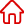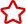﻿ POJ 3723 Conscription_C++入門知識設為首頁 加入收藏

C語言|JAVA編程
Python編程

ASP編程|PHP編程
JSP編程

MYSQL數據庫|SqlServer數據庫
Oracle數據庫|DB2數據庫
程式師世界 >> 編程語言 >> C語言 >> C++ >> C++入門知識 >> POJ 3723 Conscription

# POJ 3723 Conscription

Conscription
Time Limit: 1000MS   Memory Limit: 65536K
Total Submissions: 6325   Accepted: 2184

Description

Windy has a country, and he wants to build an army to protect his country. He has picked up N girls and M boys and wants to collect them to be his soldiers. To collect a soldier without any privilege, he must pay 10000 RMB. There are some relationships between girls and boys and Windy can use these relationships to reduce his cost. If girl x and boy y have a relationship d and one of them has been collected, Windy can collect the other one with 10000-d RMB. Now given all the relationships between girls and boys, your assignment is to find the least amount of money Windy has to pay. Notice that only one relationship can be used when collecting one soldier.

Input

The first line of input is the number of test case.
The first line of each test case contains three integers, N, M and R.
Then R lines followed, each contains three integers xi, yi and di.
There is a blank line before each test case.

1 ≤ N, M ≤ 10000
0 ≤ R ≤ 50,000
0 ≤ xi < N
0 ≤ yi < M
0 < di < 10000

Output

For each test case output the answer in a single line.
Sample Input

2

5 5 8
4 3 6831
1 3 4583
0 0 6592
0 1 3063
3 3 4975
1 3 2049
4 2 2104
2 2 781

5 5 10
2 4 9820
3 2 6236
3 1 8864
2 4 8326
2 0 5156
2 0 1463
4 1 2439
0 4 4373
3 4 8889
2 4 3133
Sample Output

71071
54223

```#include<iostream>
#include<cstdio>
#include<cstring>
#include<algorithm>
#include<cmath>
using namespace std;
int n,m;
struct node
{
int x,y,s;
}e;
int f;
int find(int x)
{
return (f[x]==x? x:f[x]=find(f[x]));
}
bool cmp(node s, node v)
{
return s.s>v.s;
}
void krusical(int p)
{
int i,j,ans=0;
for (i=0; i<p; i++)
{
int x=find(e[i].x);
int y=find(e[i].y);
if (x!=y)
{
ans+=e[i].s;
f[x]=f[y];
}
}
cout<<10000*(n+m)-ans<<endl;
}
int main ()
{
int i,j,t,r,s;
cin>>t;
while(t--)
{
int p=0;
cin>>n>>m;
for (i=0; i<=n+m; i++)
f[i]=i;
cin>>r;
while(r--)
{
scanf("%d%d%d",&e[p].x,&j,&e[p].s);
e[p++].y=j+n;
}
sort(e,e+p,cmp);
krusical(p);
}
return 0;
}```